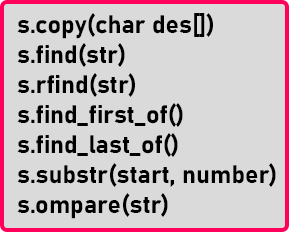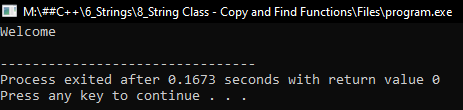# Copy and Find Functions of String Class in C++

## Copy and Find Functions of String Class in C++

In this article, I am going to discuss the Copy and Find Functions of String Class in C++ Language with examples. Please read our previous article, where we discussed Replace and Swap Functions of String Class in C++ with examples.

##### C++ String Class – Copy and Find Functions:Here we have a list of functions. Let’s see all of them one by one.

##### copy() Function of String Class in C++

Already we have shown you a string copy function that is ‘strcpy’ that works upon array. This function is similar to that function. So, it will copy a string in a character array. It will not create a new string but it will copy that in the character array. Let’s suppose we have a string and a character type array,

string str = “Welcome”;
char arr;

To copy “welcome” into the char array ‘arr’, we will write,

str.copy(arr, str.length());

This function takes 2 parameters that are first is the array in which we are copying and the second is the no. of character which we want to copy. In this case, we want to copy the whole string ‘str’ so we have given ‘str.length()’ as the second parameter. Now let us use this function in the C/C++ program:

##### Program for copy() Function of String Class in C++
```#include <iostream>
#include <string>
using namespace std;
int main()
{
string str = "Welcome";
char arr;
str.copy (arr, str.length ());
cout << arr << endl;
}
```
###### Output:If we want to copy only some characters then after that index, we have to give the null character to the char array.

Arr = “\0”;

Otherwise, the compiler will show some garbage values. Next function is find() function.

##### find() Function of String Class in C++:

This is used for finding the occurrence of a string inside the main string or a character also. For example, if there is a string,

string str = “How are you?”;
str.find(“are”);

This function will return the index of ‘are’ in the main string. So, it will return ‘4’ because ‘are’ is present at the index of ‘4’. So, it will find the occurrence of a given string from the right side.

##### rfind() Function of String Class in C++:

This function finds the occurrence from the left-hand side in the array. Suppose we have a string,

string str = “Hello”;
str.find(“o”);
str.rfind(“o”);

‘find()’ will return ‘4’ and ‘rfind()’ will return ‘0’. Here the difference between the two functions is that the ‘find’ function will look for the occurrence from the left side in the array and the ‘rfind’ function will look for the occurrence from the right side of the array.

If we give a string or word or a character that is not present in the main string then both the function ‘find’ or ‘rfind’ will return either -1 or the largest index of the main string.

So, what these functions will return? It depends on the implementation; it may change from compiler to compiler. If an index is larger than the length of the string this means that a string or a letter is not present inside the string. Let us look at the program for this.

##### Case 1: if the given string is present in the main string.
```#include <iostream>
#include <string>
using namespace std;
int main()
{
string str = "program";
cout << "find(): " << str.find ('g') << endl;
cout << "rfind(): " << str.rfind ('g') << endl;
}
```
###### Output:##### Case 2: if the given string is not present in the main string.
```#include <iostream>
#include <string>
using namespace std;
int main()
{
string str = "program";
cout << "find(): " << str.find ('s') << endl;
cout << "rfind(): " << str.rfind ('s') << endl;
}
```
###### Output:Now let us look at the next function.

##### find_first_of():

This will find the occurrence of character from the left-hand side of the string.

string str = “Hello world”;

Now if we write “str.find_first_of(‘l’)” then it will return ‘2’ as it will search for ‘l’ from the left-hand side of the array.

##### find_last_of():

This will find the occurrence of the character from the right-hand side of the string. There is another version of these function which is

str.find_last_of(‘l’, 3)

We can pass the starting index from where we want to search as the parameter. Now let us see both these functions in the program.

##### Program for find_first_of() and find_last_of() functions
```#include <iostream>
#include <string>
using namespace std;
int main()
{
string str = "program";
cout << "find_first_of(): " << str.find_first_of ('r') << endl;
cout << "find_last_of(): " << str.find_last_of ('r') << endl;
}
```
###### Output: